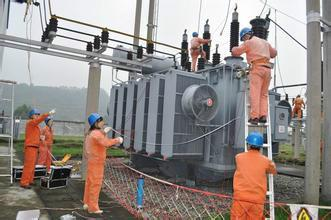Technology Literature

# What is voltage, current, power, power factor, phase and frequency?

1.Voltage: Also known as potential difference or potential difference, it is a physical quantity that measures the energy difference of a unit charge in an electrostatic field due to different potentials. This concept is similar to "water pressure" caused by high and low water levels. It should be pointed out that the word "voltage" is generally only used in circuits, while "potential difference" and "potential difference" are generally used in all electrical phenomena.

2.Current: The amount of charge passing through the cross-section of a conductor per unit time is called current, which is represented by the symbol I, the unit is ampere, and the letter "A" is represented.

3. Power: refers to the work done by an object in unit time, that is, power is a physical quantity that describes the speed of work. The amount of work is fixed, and the shorter the time, the greater the power value. The formula for finding power is power = work/time.

4.Power factor: In an AC circuit, the cosine of the phase difference (Φ) between the voltage and current is called the power factor, which is represented by the symbol cosΦ. In value, the power factor is the ratio of active power to apparent power.

5.Phase: Phase is a physical quantity that reflects the state of alternating current at any moment. The magnitude and direction of alternating current change over time. For example, sinusoidal alternating current, its formula is i=Isin2πft. i is the instantaneous value of the alternating current, I is the maximum value of the alternating current, f is the frequency of the alternating current, and t is the time. Over time, the AC current can go from zero to a maximum value, from a maximum value to zero, and again from zero to a negative maximum value, and from a negative maximum value to zero. In the trigonometric function, 2πft is equivalent to the angle, which reflects the state of the alternating current at any time, whether it is increasing or decreasing, whether it is positive or negative, and so on. Therefore, 2πft is called the phase, or the phase.

6.Frequency: Frequency is the number of times the vibration is completed per unit time, and is a quantity that describes the frequency of the reciprocating motion of a vibrating object. It is usually expressed by the symbol f or v, and the unit is second-1. To commemorate the contribution of the German physicist Hertz, people named the unit of frequency as

7. Hertz: referred to as "Hertz". Every object has an amplitude-independent frequency determined by its own properties, called the natural frequency. The concept of frequency is not only used in mechanics and acoustics, but also in electromagnetism and radio technology. The number of times the alternating current completes periodic changes per unit time is called the frequency of the current.